# Differential entropy

The formal analogue of the concept of entropy for random variables having distribution densities. The differential entropy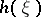of a random variabledefined on some probability space, assuming values in an-dimensional Euclidean spaceand having distribution density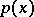,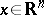, is given by the formulawhereis assumed to be equal to zero. Thus, the differential entropy coincides with the entropy of the measurewith respect to the Lebesgue measure, where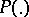is the distribution of.

The concept of the differential entropy proves useful in computing various information-theoretic characteristics, in the first place the mutual amount of information (cf. Information, amount of)of two random vectorsand. If,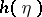and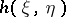(i.e. the differential entropy of the pair) are finite, the following formula is valid: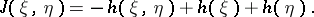The following two properties of the differential entropy are worthy of mention: 1) as distinct from the ordinary entropy, the differential entropy is not covariant with respect to a change in the coordinate system and may assume negative values; and 2) let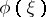be the discretization of an-dimensional random variablehaving a density, with steps of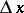; then for the entropythe formula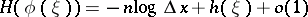is valid as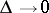. Thus,as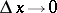. The principal term of the asymptotics of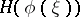depends on the dimension of the space of values of. The differential entropy defines the term next in order of the asymptotic expansion independent ofand it is the first term involving a dependence on the actual nature of the distribution of.